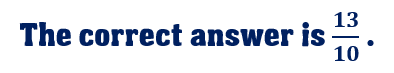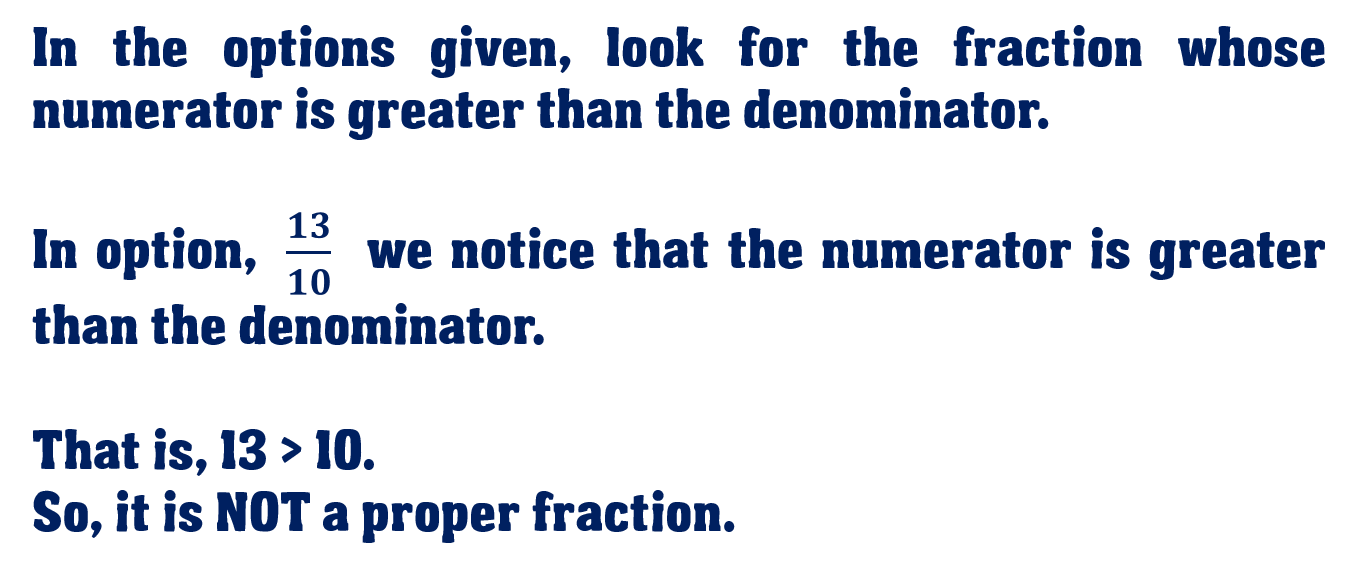1
visibility

Which one of the following is NOT a proper fraction?

• \$latex \dfrac{13}{10}\$

• \$latex \dfrac{16}{20}\$

• \$latex \dfrac{11}{18}\$

• \$latex dfrac{9}{12}\$Please Note:

A proper fraction is a fraction whose numerator (the top number) is less than the denominator (the bottom number).

An improper fraction is a fraction whose numerator (the top number) is greater than the denominator (the bottom number).

Solution: